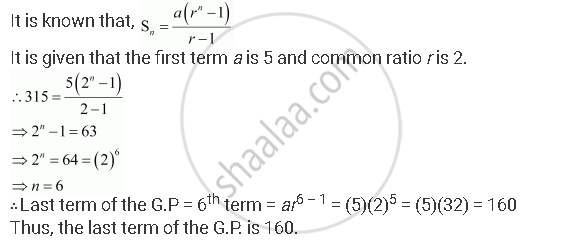CBSE (Arts) Class 11CBSE
Share
Notifications

View all notifications
Books Shortlist
Your shortlist is empty

# The Sum of Some Terms of G.P. is 315 Whose First Term and the Common Ratio Are 5 and 2, Respectively. Find the Last Term and the Number of Terms. - CBSE (Arts) Class 11 - Mathematics

Login
Create free account

Forgot password?
ConceptGeometric Progression (G. P.)

#### Question

The sum of some terms of G.P. is 315 whose first term and the common ratio are 5 and 2, respectively. Find the last term and the number of terms.

#### Solution

Let the sum of n terms of the G.P. be 315.Is there an error in this question or solution?

#### APPEARS IN

NCERT Solution for Mathematics Textbook for Class 11 (2018 to Current)
Chapter 9: Sequences and Series
Q: 8 | Page no. 199
Solution The Sum of Some Terms of G.P. is 315 Whose First Term and the Common Ratio Are 5 and 2, Respectively. Find the Last Term and the Number of Terms. Concept: Geometric Progression (G. P.).
S